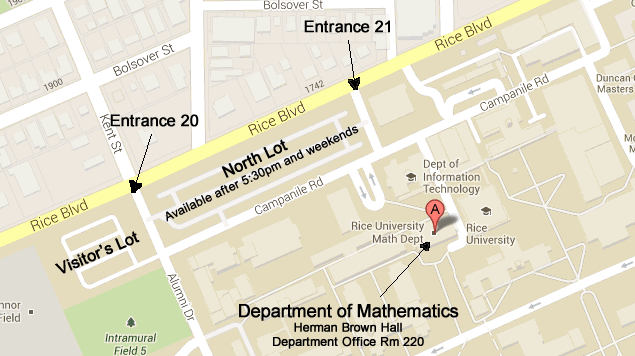### Useful courses for students interested in going into math education

• Linear Algebra (Math 354/355)
• Analysis I (Math 321)
• Algebra I (Math 356)
• Number Theory (Math 365)
• Geometry (Math 366)
• Topics in Combinatorics (Math 368)

### Useful courses for students interested in computer science

• Linear Algebra (Math 354/355)
• Analysis I (Math 321)
• Algebra I & II (Math 356 & 357)
• Number Theory (Math 365)
• Geometry (Math 366)
• Topics in Combinatorics (Math 368)
• Mathematical Cryptography (Math 323)

### Useful courses for students interested in going into economics/finance

• Linear Algebra (Math 354/355)
• Partial Differential Equations (Math 381, Math 423)
• Analysis I (Math 321)
• Analysis II (Math 322)
• Integration Theory (Math 425)
• Dynamical Systems (Math 435)
• Topology (Math 443)Large Map

Tel (713) 348-4829